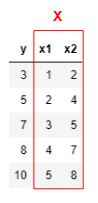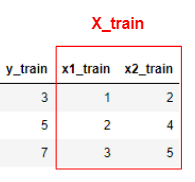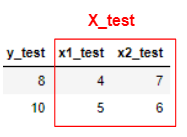# [PYTHON] Training data and test data (What are X_train and y_train?) ②

Continuing from the previous ①, we will divide the training data and test data a little more practically.

First, prepare the data.

Now there are two variables x.Thus, in machine learning, when there are two or more variables x We will combine them into one and treat them as uppercase $\ mathbf {X}$.

Next, divide it into training data and test data.

Training datatest dataThen, we will create a model formula from the training data. If this is calculated by the method of least squares, it can be calculated as follows.

y=0.9+2x_1+2.0\times10^{-16}x_2

And we will apply this to the test data.

y_{pred}=0.9+2x_{1_{test}}+2.0\times10^{-16}x_{2_{test}}=9,11

From the above, for y_test of the correct answer data I found that the y_pred I expected was roughly correct.

If there are two or more $x$ for $y$ Basically, think of training data and test data as above.

In actual machine learning, for data like this one y is the rent, x1 and x2 are the constituent elements (station walk, age, etc.) We are analyzing the data.

Also, the previous article ① and this article ② Both of them use a method called linear regression. I hope to post again in the near future.